Subsections

# Geometric Interpretation and Phase Diagrams

## Tangents and Minimization

Suppose we have a system at constant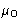. We minimize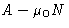.

This means we make a lineand the curve A(N). We want to minimize the distance between the line and curve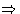we make a tangent. If the curve is above tangent, the point is locally stable, otherwise--unstable.

• One phase--one minimum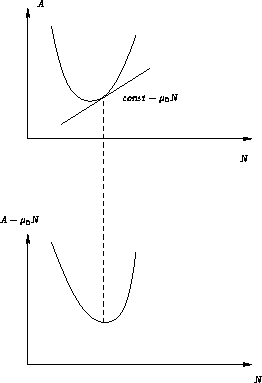• Binodal--two equal minima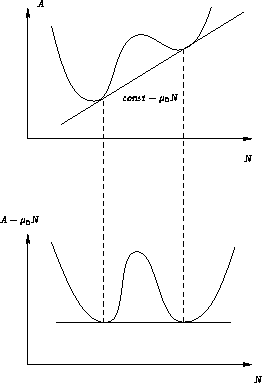• Metastable point and stable point--two unequal minima (we changedand do not have a bitangent anymore!)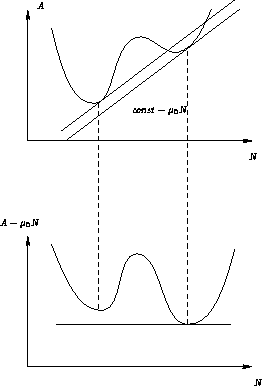• Unstable point and stable point--minimum and maximum (even greater change in)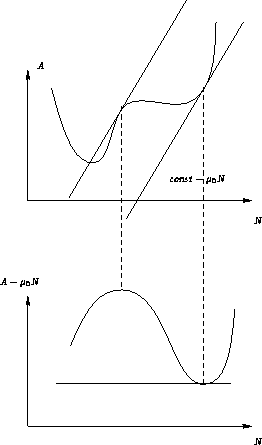Spinodal divides metastable points from stable points!

## Phase Diagrams

In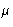-T space we have a coexistence curve: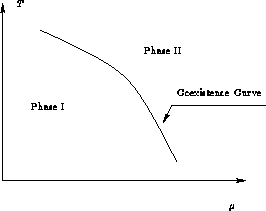In N-T space we have a coexistence region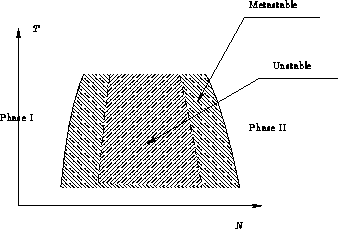Why the difference? Becauseis intensive, and N is extensive variable!

## Critical Point

Is there an ending point for coexistence curve?

Two possibilities:

1.
Free energies of two phases A1 and A2 are different curves--ending point! Liquid-solid phase transition.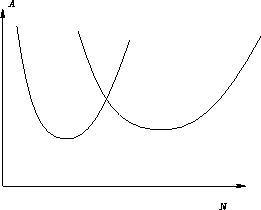2.
A1 and A2 are parts of same curve--ending point is possible! it is called critical point. Liquid-gas phase transition.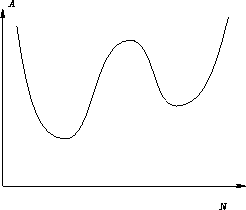Next: Gibbs Phase Rule Up: Phase Equilibria Previous: Free Energy Minima and

© 1997 Boris Veytsman and Michael Kotelyanskii
Thu Oct 2 21:02:12 EDT 1997